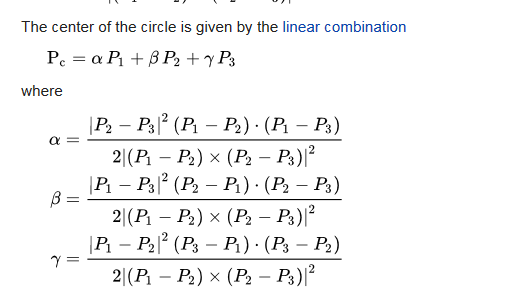# Algebraic formulas of objects

Clueid shared this question 2 years ago

Hello,

GeoGebra is an excellent tool to calculate numeric values efficiently. But how can I calculate algebraic formulas rather than numeric values?

I know commands like factor() and expand(), etc... but what I need is the algebraic formula of the dependent objects.

For example, given 3 free points, instead of calculating the numeric value of their circumcenter, I want it in terms of the 3 points coordinates: (a_1, b_1), (a_2, b_2), (a_3, b_3).2

Try the GeoGebra CAS App, eghttps://www.geogebra.org/ca...2

don't work for circumcenter21

Great! But is it possible to:

1) Make custom tools or commands in CAS? For example, a command for finding the orthocenter, or circumcenter.. etc...?

2) Take input from existing objects in graphic view. It would take a lot of time in complicated diagrams to write down the command for every construction.

Thank you!2

why in CAS? you can do a custom tool for each of them in GG without CAS

do you know the TriangleCentre( <Point>, <Point>, <Point>, <Number> ) command?2

la question porte sur le calcul littéral, il n'est pas possible en dehors du CAS2

it can be found in wikipedia (ie: the first example in first post)1

Thank you mathmagic for your reply. I just gave the circumcenter as an example. What about calculating formulas of more complicated diagrams?2

j'entends bien, de la même façon il n'y a pas besoin d'utiliser GeoGebra pour construire le triangle rectangle "3-4-5" puisqu'il est déjà construit sur Wikipédia